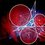# A Mathematics Section on Geometric Constructions

According to me, Geometric construction is an art that is just as mathematically demanding as the art of solving geometry problems given geometric data (viz, length, angles, collinearities etc.) that we conventionally do.

So, I propose here a new section on Geometric Construction Challenges where just like the pre-existing sections on Algebra or Geometry, we shall have problems in Constructions. Of course the current format of answering should be changed: The answer would be like to post an image of one's construction.

Example: Given the medians of a triangle are 3,4 and 5, Construct the triangle using only compasses and straightedge take as standard the unit length. Measure the circumradius and report it as the answer. Then upload your image.

Answer: First the perimeter. Points gained. Then an image of the constructed triangle with clear view of the arcs, lines used for the construction and helpful steps if required. After the image has been verified by the problem-setter, more points will be rewarded for the image! These extra points only to the first image to be posted.

Can this already be done by a member himself? If so, how? Otherwise, I think we should have such a section.... Upvote and reshare if you agree!6 years, 7 months ago

This discussion board is a place to discuss our Daily Challenges and the math and science related to those challenges. Explanations are more than just a solution — they should explain the steps and thinking strategies that you used to obtain the solution. Comments should further the discussion of math and science.

When posting on Brilliant:

• Use the emojis to react to an explanation, whether you're congratulating a job well done , or just really confused .
• Ask specific questions about the challenge or the steps in somebody's explanation. Well-posed questions can add a lot to the discussion, but posting "I don't understand!" doesn't help anyone.
• Try to contribute something new to the discussion, whether it is an extension, generalization or other idea related to the challenge.

MarkdownAppears as
*italics* or _italics_ italics
**bold** or __bold__ bold
- bulleted- list
• bulleted
• list
1. numbered2. list
1. numbered
2. list
Note: you must add a full line of space before and after lists for them to show up correctly
paragraph 1paragraph 2

paragraph 1

paragraph 2

[example link](https://brilliant.org)example link
> This is a quote
This is a quote
    # I indented these lines
# 4 spaces, and now they show
# up as a code block.

print "hello world"
# I indented these lines
# 4 spaces, and now they show
# up as a code block.

print "hello world"
MathAppears as
Remember to wrap math in $$ ... $$ or $ ... $ to ensure proper formatting.
2 \times 3 $2 \times 3$
2^{34} $2^{34}$
a_{i-1} $a_{i-1}$
\frac{2}{3} $\frac{2}{3}$
\sqrt{2} $\sqrt{2}$
\sum_{i=1}^3 $\sum_{i=1}^3$
\sin \theta $\sin \theta$
\boxed{123} $\boxed{123}$